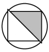CompEng

### Materially Non-Linear Finite Element Analysis Using a Scalar Damage Model

Structural failures usually occur as a result of high loading conditions. Crack initiation and growth are one of the main causes for damage. Unexpected failure of a part during the structure's lifetime can be costly for a number of reasons both financial and in terms of human life.

In this thesis a continuum damage model is implemented in the finite element program COMSOL Multiphysics. The theoretical framework is based on the thermodynamic theory of energy and material dissipation, described by a set of fundamental formulations of constitutive equations of damaged materials, development equations of the damaged state, and evolution equations of micro-structures.

A scalar damage model is introduced, in which the stiffness degradation is described by a single scalar variable d. The increase of damage variable is controlled by a damage threshold parameter κ defined as a maximum of the equivalent strain measure η obtained during the load history up to time t. This evolution of the internal damage variables is taken into account by explicitly solving the corresponding ordinary differential equation.
Numerical simulations of typical damage scenarios show the capabilities of the implemented model.

### Lehrstuhl und Betreuer

Lehrstuhl für Grundbau, Boden- und Felsmechanik
(Prof. Dr.-Ing. habil. Tom Schanz)### Betreuung

Dr.Ing. C. Becker